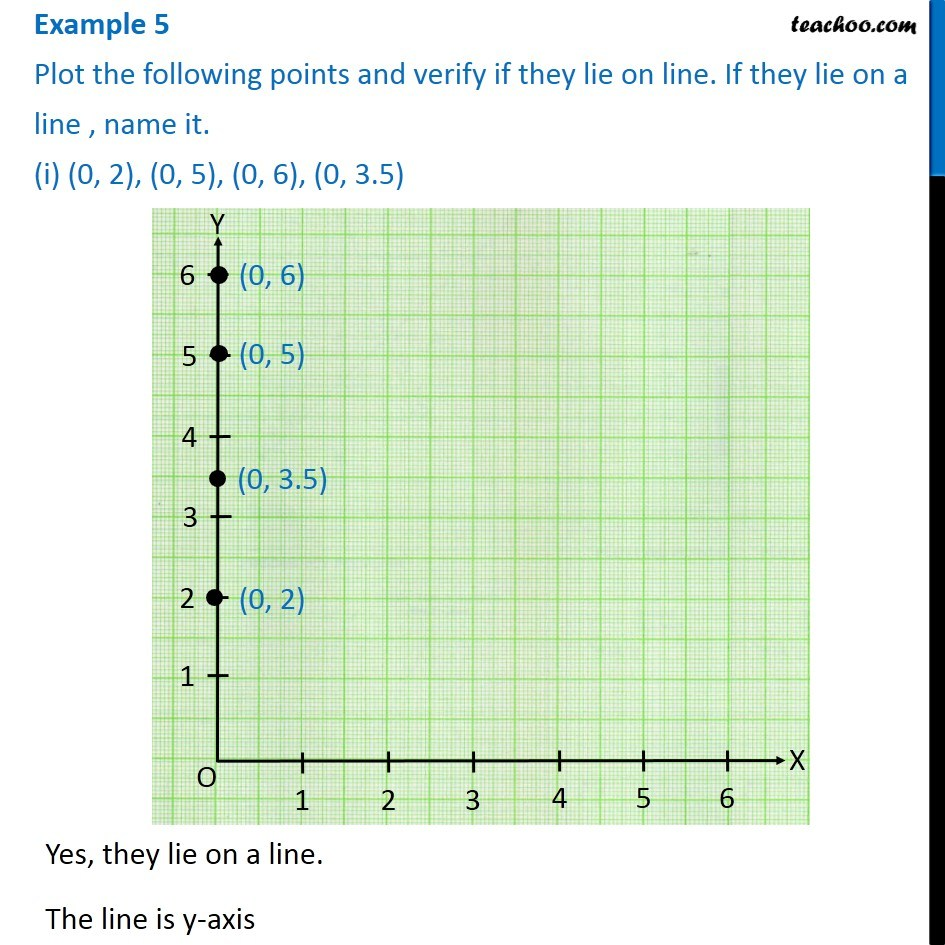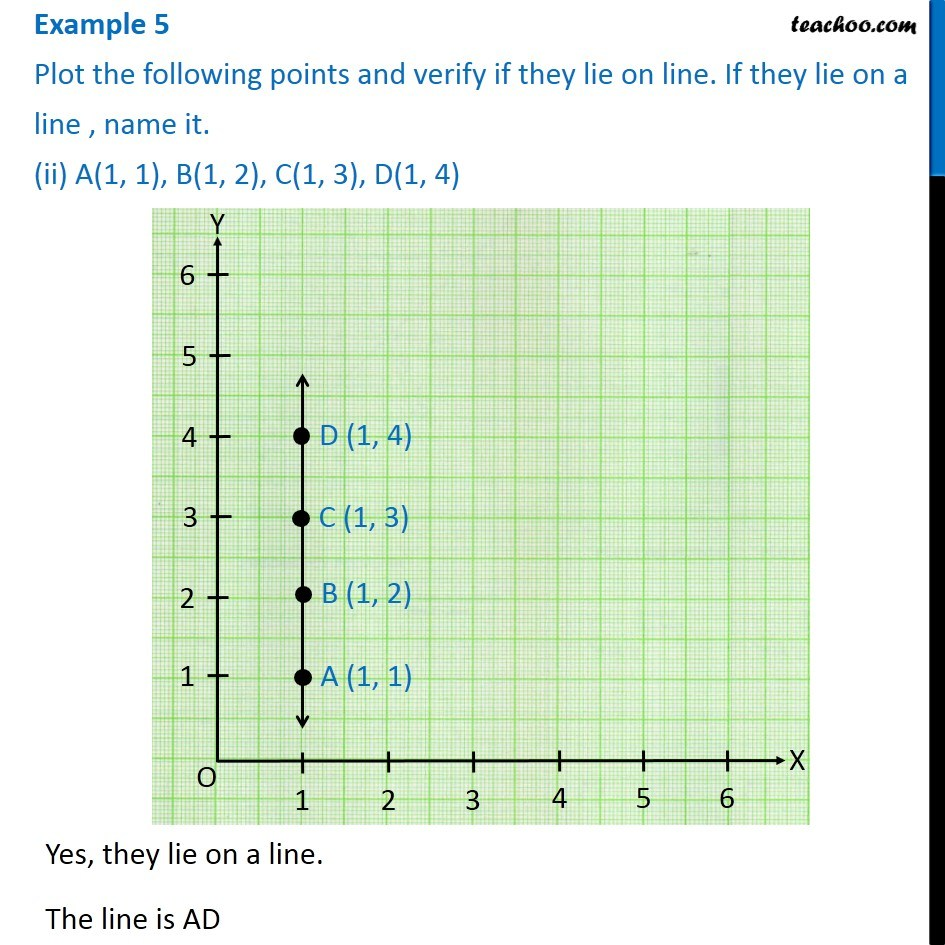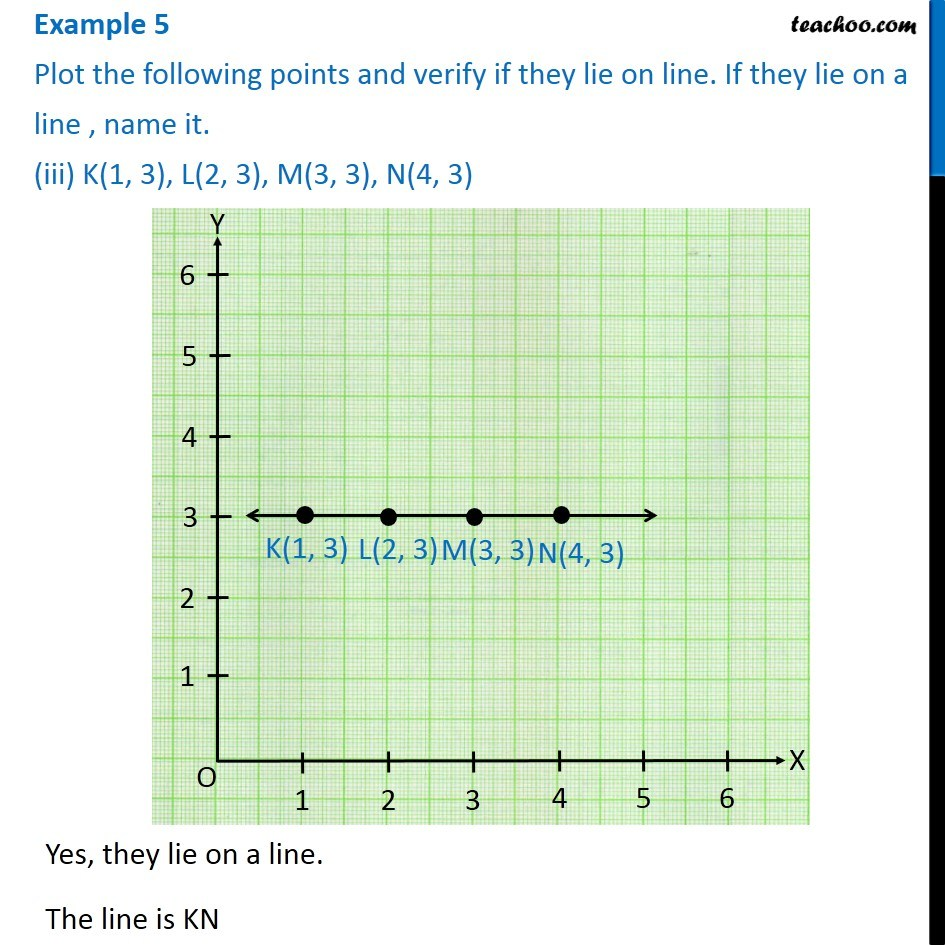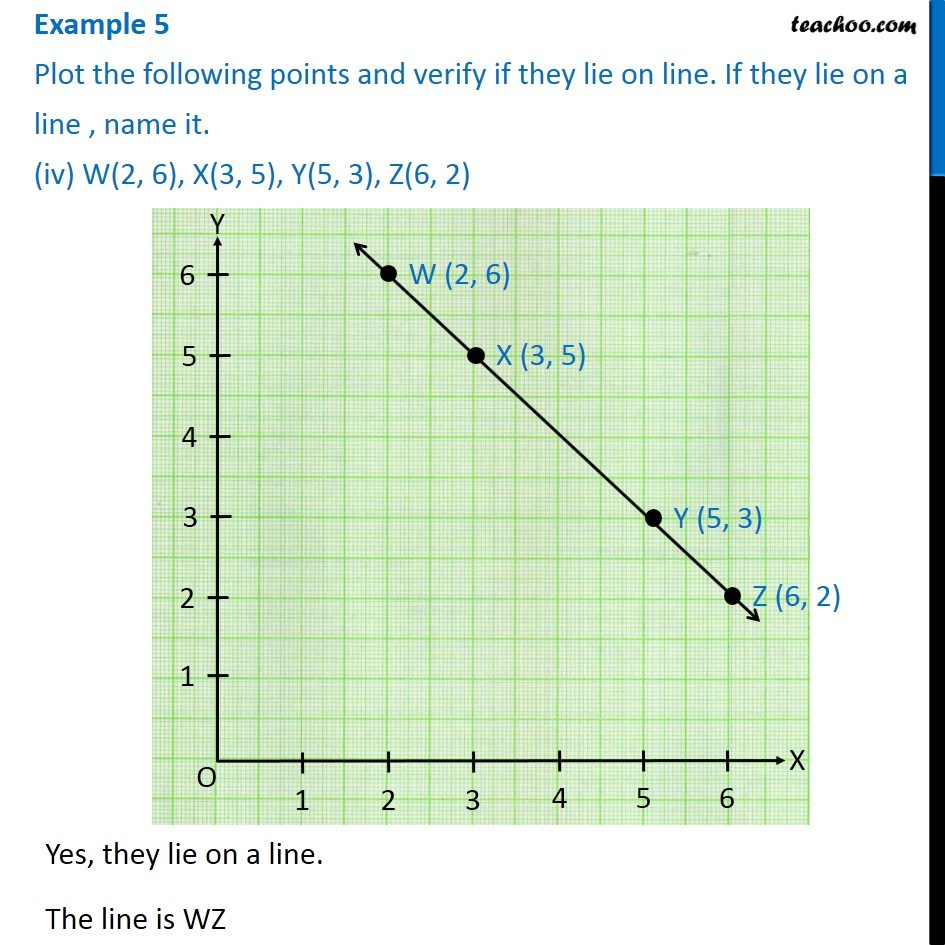Subscribe to our Youtube Channel - https://you.tube/teachoo

1. Chapter 15 Class 8 Introduction to Graphs
2. Serial order wise
3. Examples

Transcript

Example 5 Plot the following points and verify if they lie on line. If they lie on a line , name it. (i) (0, 2), (0, 5), (0, 6), (0, 3.5) Yes, they lie on a line. The line is y-axis Example 5 Plot the following points and verify if they lie on line. If they lie on a line , name it. (ii) A(1, 1), B(1, 2), C(1, 3), D(1, 4) Yes, they lie on a line. The line is AD Example 5 Plot the following points and verify if they lie on line. If they lie on a line , name it. (iii) K(1, 3), L(2, 3), M(3, 3), N(4, 3) Yes, they lie on a line. The line is KN Example 5 Plot the following points and verify if they lie on line. If they lie on a line , name it. (iv) W(2, 6), X(3, 5), Y(5, 3), Z(6, 2)Yes, they lie on a line. The line is WZ

Examples